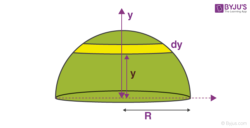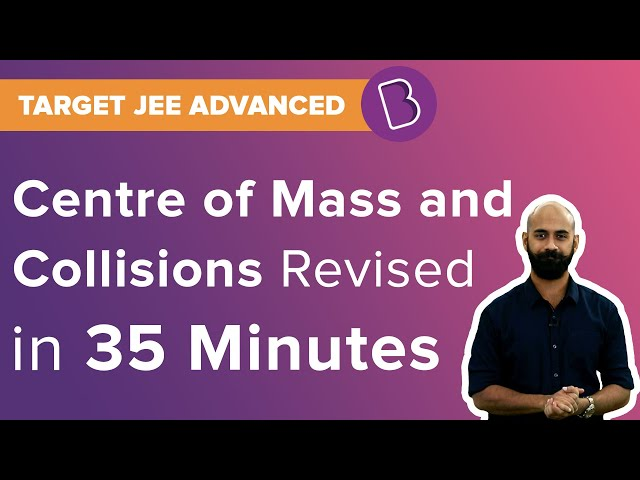Win up to 100% scholarship on Aakash BYJU'S JEE/NEET courses with ABNAT Win up to 100% scholarship on Aakash BYJU'S JEE/NEET courses with ABNAT

# Centre of Mass of Solid Hemisphere

There is a special point in a system or object, called the centre of mass that moves as if all of the mass of the system is concentrated at that point. The system will move as if an external force is applied to the object of mass M located at the centre of mass. In this article, we will determine the centre of mass of a solid hemisphere.

## How to Find Centre of Mass of Solid HemisphereWe are considering a solid hemisphere of mass M and has the radius R. The centre of mass will lie on the vertical line passing through the centre of the hemisphere, the vertical line is also the normal to the base. In order to find the centre of mass, we have to consider an element.

We are taking an elemental disc at a height h from the base of the hemisphere. The mass of the elemental disc is dM and the width is dy.

The radius of the disc is

$$\begin{array}{l}r=\sqrt{R^{2}-y^{2}}…..(1)\end{array}$$

Mass of the disc dM = (3M/2πR3) × (πr2dy)…..(2)

Substitute equa(1) in equa(2)

dM = (3M/2πR3) × π(R2 – y2)dy)

Y-coordinate of Centre of mass,yc = (1/M)ഽydM,

Here y is the y-coordinate which represents the height of the elemental disc from the base.

Putting the value of dM and calculating the centre of mass, we get

yc= (1/M)ഽy(3M/2R3) × (R2-y2)dy)

Integrating between the limits 0⟶R

$$\begin{array}{l}y_{c}=\frac{1}{M}\int_{0}^{R}\frac{3M}{R^{3}}(R^{2}-y^{2})dy\times y\end{array}$$

$$\begin{array}{l}y_{c}=\frac{3}{2R^{3}}\int_{0}^{R}{}(R^{2}y-y^{3})dy\end{array}$$

= (3/2R2)[(R4/2) – (R4/4)] = 3R/8

yc = 3R/8

Centre of Mass of the solid hemisphere, yc = 3R/8,

Here R is the radius of the hemisphere.

## Centre of Mass and Collisions## Frequently Asked Questions on Centre of Mass of Solid Hemisphere

Q1

### What is the formula for the centre of mass of a solid hemisphere?

3R/8
R is the radius of the hemisphere.

Q2

### What is the law of conservation of linear momentum of the centre of mass?

The linear momentum of the centre of mass of a system remains constant if no external force acts on the system.

Q3

### The centre of gravity of a body on the earth coincides with the centre of mass for a small object whereas for an extended object it may not. What is the qualitative meaning of small and extended in this regard?

An object whose vertical dimensions are negligible as compared to the radius of the earth is known as a small object. On the other hand, an object whose vertical dimension is not negligible as compared to the radius of the earth is known as an extended object.

Test Your Knowledge On Centre Of Mass Of Solid Hemisphere!